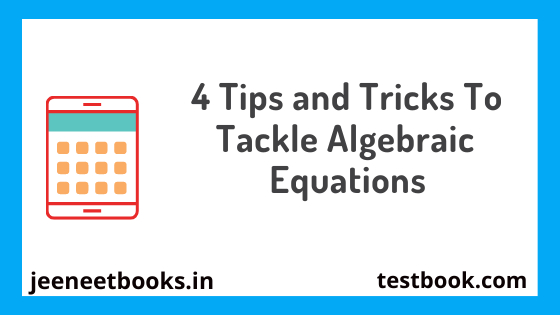# 4 Tips and Tricks To Tackle Algebraic Equations4 Tips and Tricks To Tackle Algebraic Equations

In mathematics, the algebraic equation is the most confusing factor for students where it reduces the marks of best brains. The variables, exponentials, fractions, and other parts of algebra are somewhere down the line seems a challenging task. However, having good command over basics and good mathematical concepts will allow students to easily solve algebraic equations.

In the beginning, while solving equations, there would be miscalculations and wrong answers. Also, it becomes a tedious task when the same calculation process students have to follow to get the right answer. Hence, we bought a few effective tips that streamline the solving process. Read on.

How to Solve Algebraic Equations?

### 1. Write the question

First, writing the question is the major task and a key step to initiate the solving process. Why is it relevant? It is because you can easily understand the variables and exponents of which determining value is required. After knowing all elements of the equation, start solving it. Remember, do not skip any step or use shortcuts while solving.

Follow every calculation step thoroughly to land up getting the right answer. Besides, do not ignore mathematical operations and follow the BODMAS (Brackets, Orders, Division/Multiplication, Addition/Subtraction) rule while solving equations. To get the correct answer, follow this first step and get an accurate answer.

### 2. Solve the bracket first

As mentioned above, students need to solve the bracket value first. For instance, the equation is 30x+23y+3(2x+9y)=? So, in this case, solve 3(2x+9y). As the BODMAS rule says, this part first comes to the bracket and then another mathematical operation. After solving this part, then solve the rest of the equation.

Any kind of wrong approach or miscalculation will give you the wrong answer. Also, there is no need to make the solving process complicated. Follow easy calculation measures and a simple approach. Not just in algebra, but solving double line graph questions also students sometimes follow the complicated approach. Thus, they land up solving the inappropriate solution. Therefore, it is advisable to follow the simple and right approach for calculation.

3. Keep an eye on square

See this equation 3x2 + (2y+6x)2 =? So, as per the BODMAS rule, you will solve the bracket first. That is a good thing! But one square factor is also there. That square power means you have to multiply the same number by itself. Hence, in the given equation, first, find out the square of 2x+6y and then start solving the equation.

To find the square of the equation, follow the simple multiplication method. Remember that multiplication of algebraic equations is easy. There is no need for calculators. Have good multiplication skills and get the right answer.

### 4. Find the value of x

Now, if the question is 3(x+7) =30 and you have to find the value of 'x's, then how to solve it? Here comes the division rule into existence where '3' will be multiplied by x and '7'. So, what did you get? 3x+21=30. As per the basic mathematical rule, integers will come on either side of the equation, which means the next step will be 3x=30-21 which gives 3x=9.

The final approach is to divide the 9 and 3 and get the value of 'x's. In our equation, the value of x is 3. Hence, here is the final answer that will secure your grades. Follow the same approach in all kinds of algebraic equations. However, we shared the example of 'x'; you may get the 'y' and 'z' or any other alphabetic characters in equations. But, the same approach is required to get the value of every variable. Hence, do not ignore these key steps.

### Related Tags

algebra by 25 trick algebra short tricks pdf algebraic equations examples with answers algebra tricks for competitive exams algebra shortcuts how to solve algebraic expressions algebra tricks for ssc cgl

### 1 Response to "4 Tips and Tricks To Tackle Algebraic Equations"

1.## Below Post Ads

For Removal of any book links. Contact us : zaphieyr4you@gmail.com. Check out Disclaimer Page for More Information.